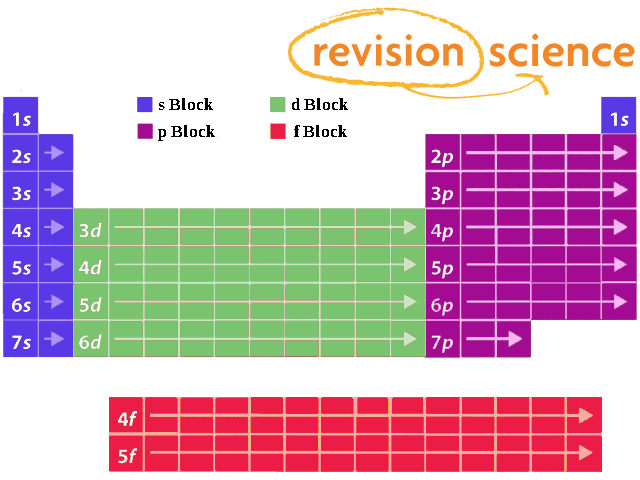## Title

Shells and Subshells
Shells

Electrons orbit the nucleus of an atom at different ranges, called shells.
Each shell has a different energy level, increasing the further it is from the nucleus.

Each energy level is given a number called the principal quantum number, n. The closest shell has a value of n=1. The next shell has a value of n=2, etc.

The maximum number of electrons possible in the first four energy levels are:

 n= Shell Maximum Number of Electrons 1 1st Shell 2 2 2nd Shell 8 3 3rd Shell 18 4 4th Shell 32

Using the above you can work out the maximum number of electrons that can occupy a shell is 2n2.
Electrons are placed into available shells, starting with the lowest energy level. Each shell must be full before the next starts to fill. This model breaks down at the n=3 shell because each shell has subshells.

Subshells

There are 4 subshells, s, p, d, and f. Each subshell can hold a different number of electrons.

 SubShell Electrons s 2 p 6 d 10 f 14

The n number determines how many of the subshells make up the shell. For example, the 1st shell is made up of 1 subshell, s. It can therefore contain only 2 electrons.
The 2nd shell is made up of 2 subshells, s and p. It can therefore contain 2+6=8 electrons.

A complete table for the first four shells looks like:

 Shell Subshell Total Number of Electrons in Shell 1st Shell 1s 2 2nd Shell 2s, 2p 2 + 6 = 8 3rd Shell 3s, 3p, 3d 2 + 6 + 10 = 18 4th Shell 4s, 4p, 4d, 4f 2 + 6 + 10 + 14 = 32

The number before each subshell specifies which shell it belongs to.
As an example, Lithium has 3 electrons. 2 will first fill up the 1st shell in subshell 1s. The remaining electron will appear in the second shell in the 2s subshell.

You can write the full electron configuration in terms of subshells.
Going back to the above example, Lithium is 1s22s1 (1s has 2 electrons, 2s has 1 electron).

Similar electron configurations within a group of the Periodic Table can be emphasised with a simpler representation in terms of the previous noble gas.

Lithium 1s22s1 can be simplified to [He]2s1 as Helium (He) has an electron configuration of 1s2.

Note: subshells have different energy levels which can confuse the order they fill.

Subshells and Periodic TableElements are grouped in blocks that refer to the subshell that contains the highest energy electron.

For example, any element in the row 3d will have it's highest energy electron in sub-shell d of the 3rd shell, whereas an element in row 4d will have the highest energy electron in sub-shell d of the 4th shell.